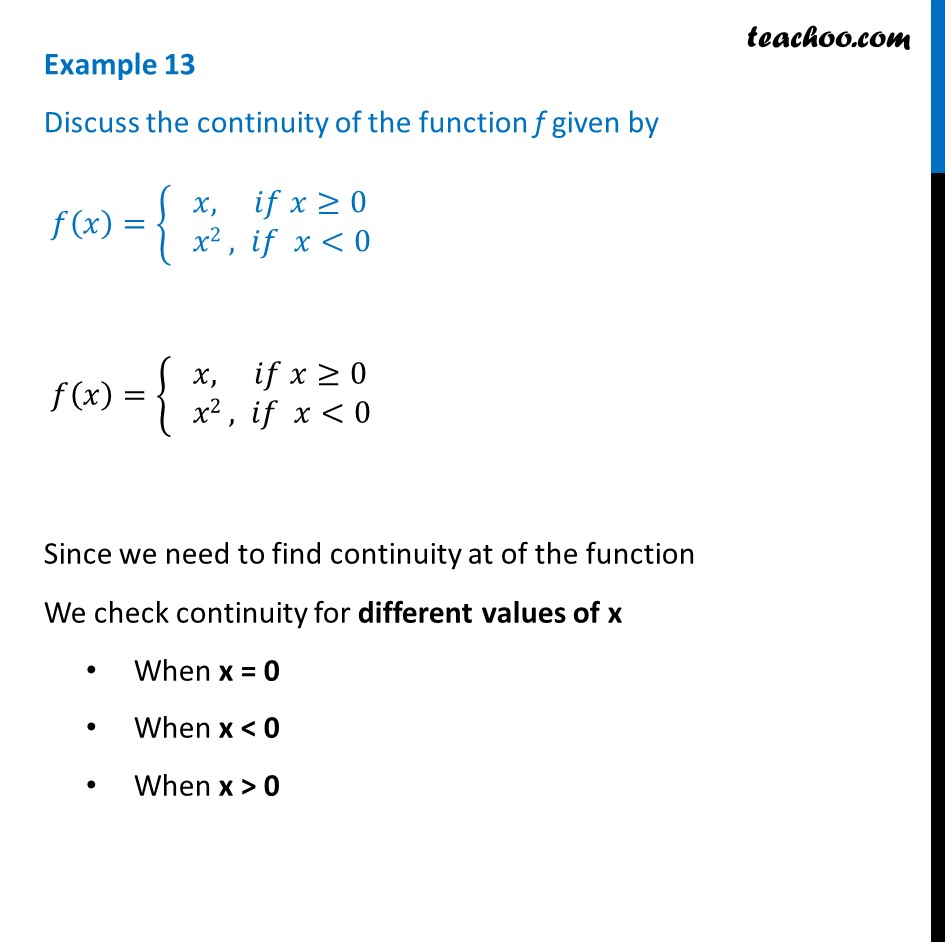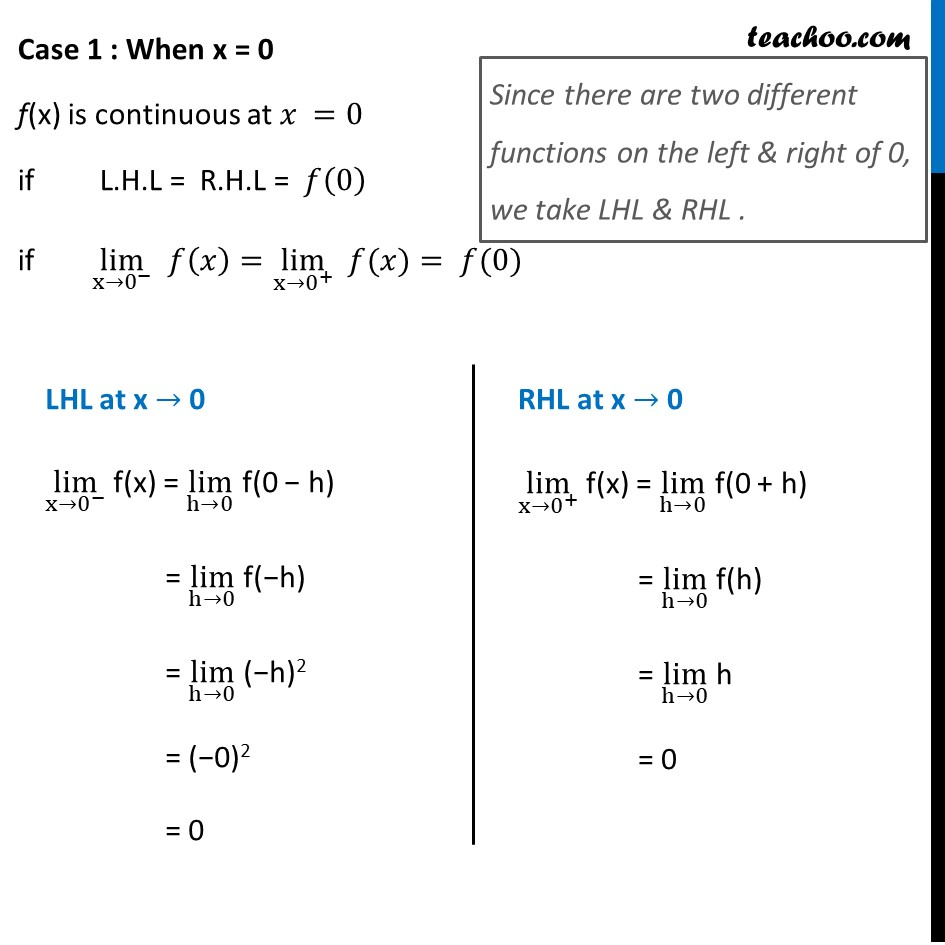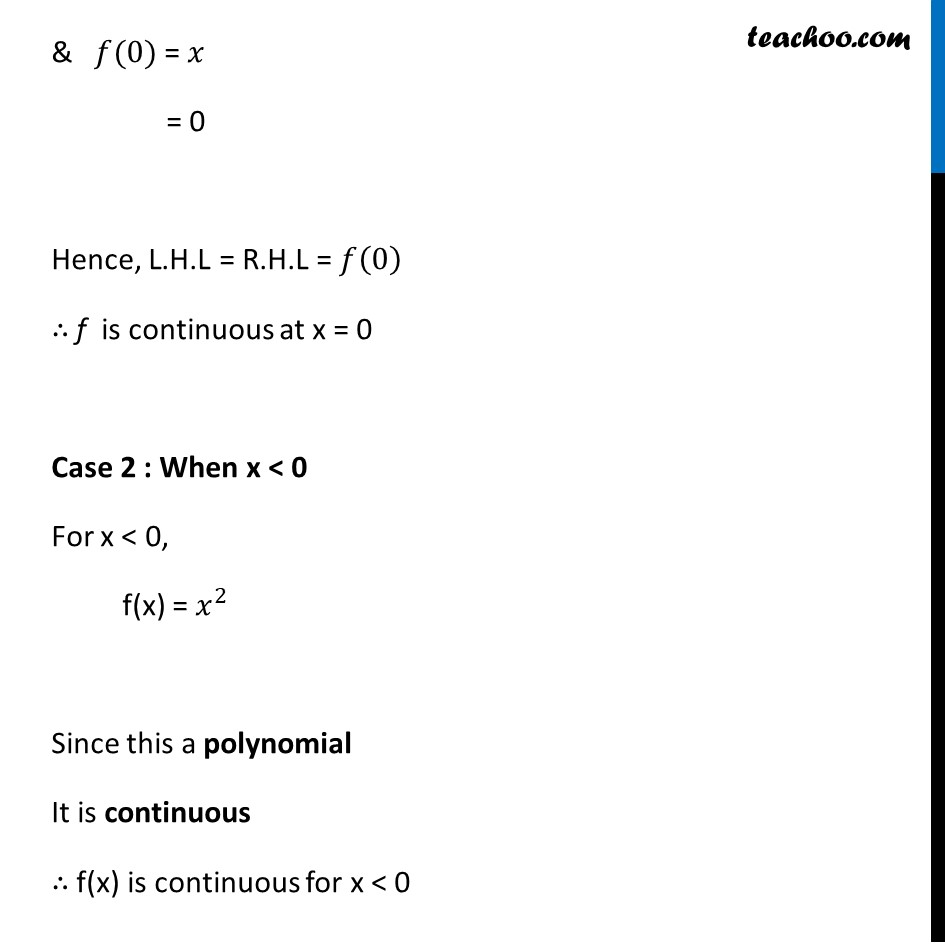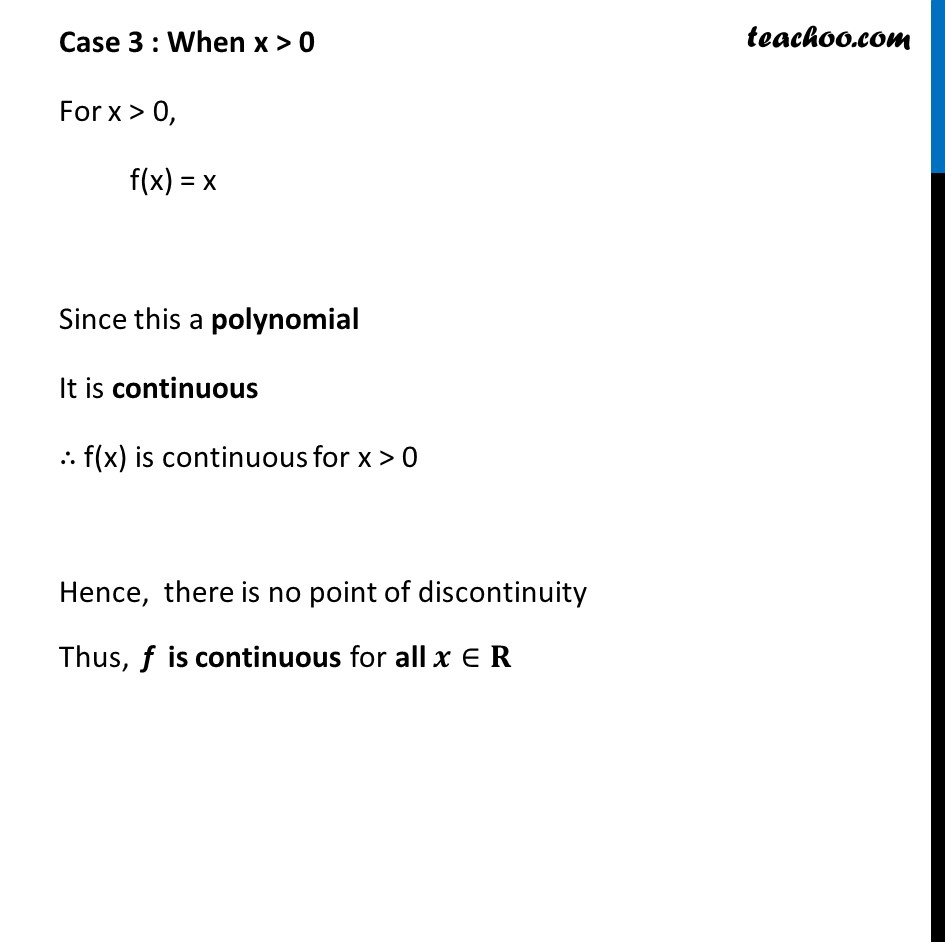Examples

Chapter 5 Class 12 Continuity and Differentiability
Serial order wiseGet live Maths 1-on-1 Classs - Class 6 to 12

### Transcript

Example 13 Discuss the continuity of the function f given by 𝑓(𝑥)={█(& 𝑥, 𝑖𝑓 𝑥≥[email protected]& 𝑥2 , 𝑖𝑓 𝑥<0)┤ 𝑓(𝑥)={█(& 𝑥, 𝑖𝑓 𝑥≥[email protected]& 𝑥2 , 𝑖𝑓 𝑥<0)┤ Since we need to find continuity at of the function We check continuity for different values of x When x = 0 When x < 0 When x > 0 Case 1 : When x = 0 f(x) is continuous at 𝑥 =0 if L.H.L = R.H.L = 𝑓(0) if lim┬(x→0^− ) 𝑓(𝑥)=lim┬(x→0^+ ) " " 𝑓(𝑥)= 𝑓(0) Since there are two different functions on the left & right of 0, we take LHL & RHL . LHL at x → 0 lim┬(x→0^− ) f(x) = lim┬(h→0) f(0 − h) = lim┬(h→0) f(−h) = lim┬(h→0) (−h)2 = (−0)2 = 0 RHL at x → 0 lim┬(x→0^+ ) f(x) = lim┬(h→0) f(0 + h) = lim┬(h→0) f(h) = lim┬(h→0) h = 0 & 𝑓(0) = 𝑥 = 0 Hence, L.H.L = R.H.L = 𝑓(0) ∴ f is continuous at x = 0 Case 2 : When x < 0 For x < 0, f(x) = 𝑥^2 Since this a polynomial It is continuous ∴ f(x) is continuous for x < 0 Case 3 : When x > 0 For x > 0, f(x) = x Since this a polynomial It is continuous ∴ f(x) is continuous for x > 0 Hence, there is no point of discontinuity Thus, f is continuous for all 𝒙∈𝐑